Gram-Schmidt orthogonalization and approximation

We want to find the polynomial of degree 4 that is the best approximation to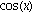on the interval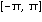.  To do this we first take the standard basis for polynomials, {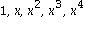} and use Gram-Schmidt to construct an orthonormal basis. While we could do this more efficiently using built-in commands of maple, we will just use maple as a calculator.

First, we define our inner product as a function on two functions.  Our inner product is the usual one, that is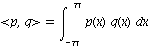.   Note that ||p-q|| is small precisely when the values of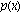are close to those of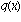for all x in the interval.

For simplicity, we'll operate on expressions inrather than general functions.

 > InnerProd:=(p,q) -> int(p*q, x=-Pi..Pi);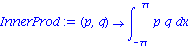We'll also want to compute the norm of our functions.  However, Norm is a maple built-in function, so we'll be a little more formal and call Norm by his full name.

 > Norman:=p->sqrt(InnerProd(p,p));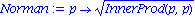Now we do Gram-Schmidt to construct an orthonormal basis (with respect to this inner product) for polynomials of degree 4.

The first element of this basis is the constant function.  However, we need to normalize it to be of unit length in our norm.

 > f := 1/Norman(1);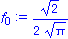Next, we confirm that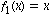is orthogonal to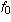.  Since we want an orthonormal basis, we still want to divide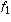by its length

 > InnerProd(x,f);> f:= x/Norman(x);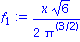Notice that while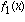is orthogonal to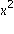,is not.

 > InnerProd(x^2,f);> InnerProd(x^2,f);This means we have to use Gram-Schmidt to find a function in the span of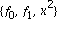which is orthogonal to bothand.  Note that sinceandare already normalized, we don't have to divide by their lengths.

 > ftemp:= x^2 - InnerProd(x^2,f)*f - InnerProd(x^2,f)*f; f  := collect(ftemp/Norman(ftemp),x);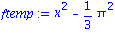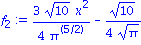Now we play the same game with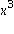and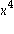.  Note that if you were working these integrals out by hand, you'd quickly realize that the even powers are orthogonal to the odd ones.

 > ftemp:= x^3 - InnerProd(x^3,f)*f - InnerProd(x^3,f)*f - InnerProd(x^3,f)*f: f  := collect(ftemp/Norman(ftemp),x);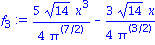> ftemp:= x^4 - InnerProd(x^4,f)*f - InnerProd(x^4,f)*f - InnerProd(x^4,f)*f - InnerProd(x^4,f)*f: f  := collect(ftemp/Norman(ftemp),x);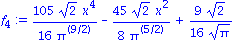So, we now have an orthonormal basis for fourth degree polynomials on.  Just in case you are disturbed by all the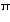s and square roots, let's also write their approximate values, and also draw their graphs.

 > OrthoBasis:=[f,f,f,f,f];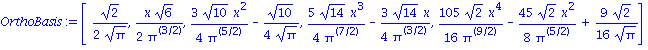> evalf(OrthoBasis,4);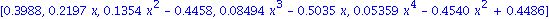> plot(OrthoBasis,x=-Pi..Pi, color=[black,red,blue,green,brown],thickness=2);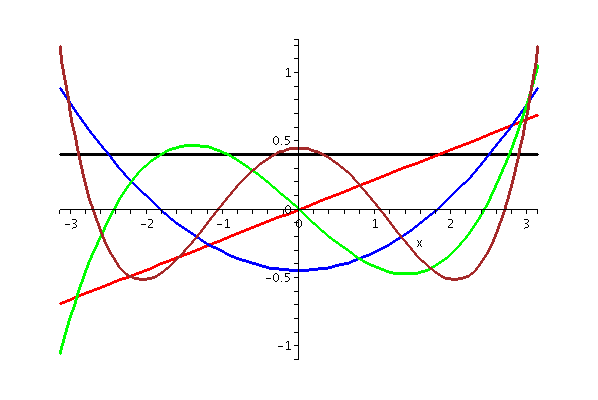The orthonormal basis we have constructed is closely related to the Legendre polynomials, one of several families of orthogonal polynomials.

Now we can turn to our minimization problem, which becomes easy with an orthonormal basis.

Recall that the minimum with respect to our norm is just the projection of the target function onto the subspace spanned by the basis.  With an orthogonal basis, this is trivial since it is just a sum of inner products times each basis element.
While we know that the inner product ofwith,, and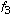is zero, we write them anyway.

 > approx := collect(  InnerProd(cos(x),f)*f + InnerProd(cos(x),f)*f + InnerProd(cos(x),f)*f + InnerProd(cos(x),f)*f + InnerProd(cos(x),f)*f, x); evalf(approx);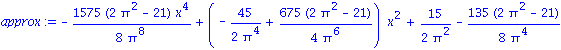The fit is pretty good, differing by less than .07 on the whole interval.

 > plot([cos(x),approx], x=-Pi..Pi, color=[red, green], thickness=2);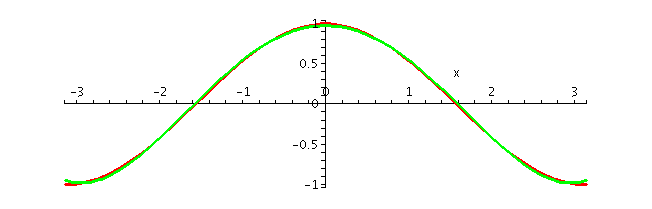For comparison, let's look at the Taylor polynomial for the cosine on the same interval.  Note that while the Taylor polynomial fits much better for small values, over the whole interval it is much worse.

 > tailor:=convert(taylor(cos(x),x,5),polynom);

 > plot([cos(x), tailor], x=-Pi..Pi, color=[red, blue], thickness=2);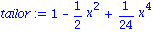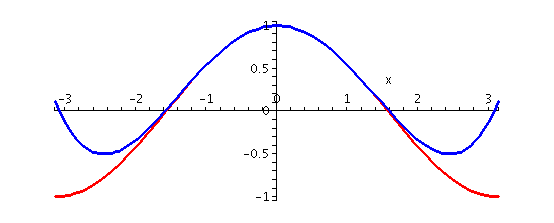More explictly, we can compare the norms of the differences, and see the dramatic difference.  We also show a plot of the two differences on the same axes.

 > evalf(Norman(cos(x)-approx)); evalf(Norman(cos(x)-tailor));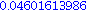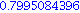> plot([cos(x)-approx, cos(x)-tailor], x=-Pi..Pi, color=[green,blue], thickness=2);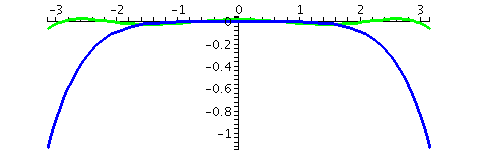Just to emphasize the ease of solving the minimization problem once the basis is in hand, here are a couple more examples.

Naturally, since our basis was constructed specifically for the interval, we must work there. However, it would be not hard to adapt this same basis to work on any interval.

In order to avoid lots of typing, we write a Maple function that computes the inner product for us, and another that shows us the graphs.

 > MakeApprox:=(f,basis)->collect(add(InnerProd(f,basis[i])*basis[i], i=1..nops(basis)),x): ShowApprox:=proc(f,basis)  local a;  a:=MakeApprox(f,basis);  print(a);  print(evalf(a), " norm of difference=",evalf(Norman(a-f)));  plot([f, a], x=-Pi..Pi, color=[red, green], thickness=2); end:

First, just to check, we apply it to the same problem we just solved.

 > ShowApprox(cos(x),OrthoBasis);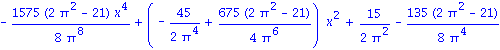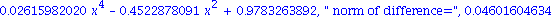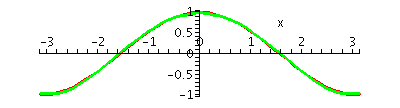Now we will try several other functions, just to show off.  First, sin(x), which doesn't do as well (we'd need to add a fifth degree polynomial to our basis for a good fit.)

 > ShowApprox(sin(x),OrthoBasis);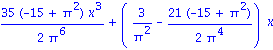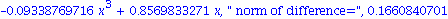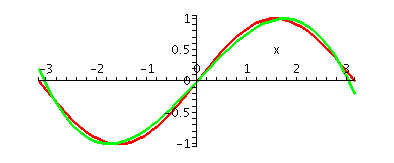> ShowApprox(cos(1+x)-x,OrthoBasis);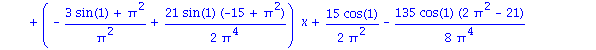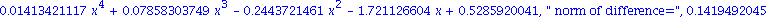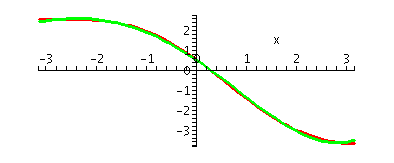> ShowApprox(exp(x)*cos(x),OrthoBasis);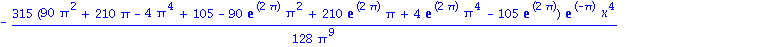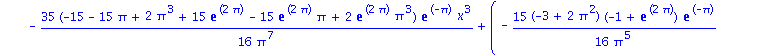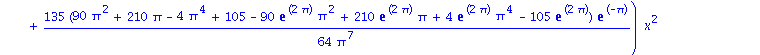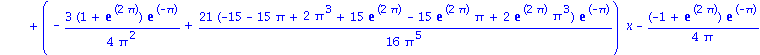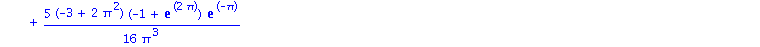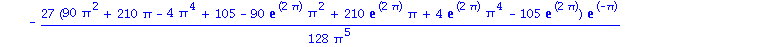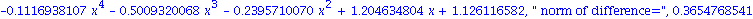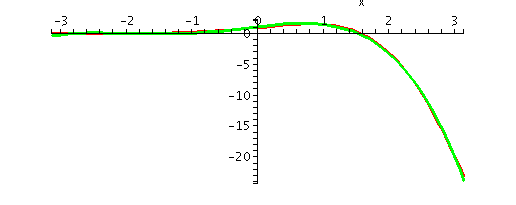Scott Sutherland May 9, 2005